# Unit 7 Stoichiometry I Stoichiometry Calculations Proportional Relationships

• Slides: 31Unit 7 - Stoichiometry I. Stoichiometry CalculationsProportional Relationships 2 1/4 c. flour 1 tsp. baking soda 1 tsp. salt 1 c. butter 3/4 c. sugar 3/4 c. brown sugar 1 tsp vanilla extract 2 eggs 2 c. chocolate chips Makes 5 dozen cookies. b. I have 5 eggs. How many cookies can I make? 5 eggs 5 dozen 2 eggs Ratio of eggs to cookies = 12. 5 dozen cookies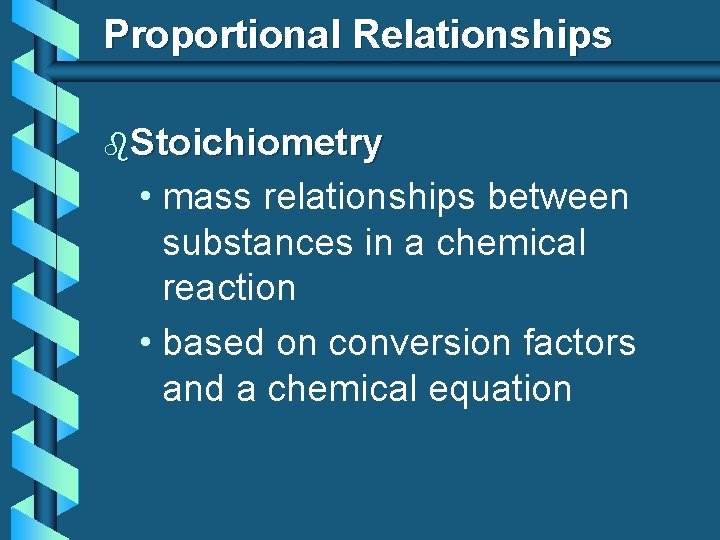Proportional Relationships b. Stoichiometry • mass relationships between substances in a chemical reaction • based on conversion factors and a chemical equation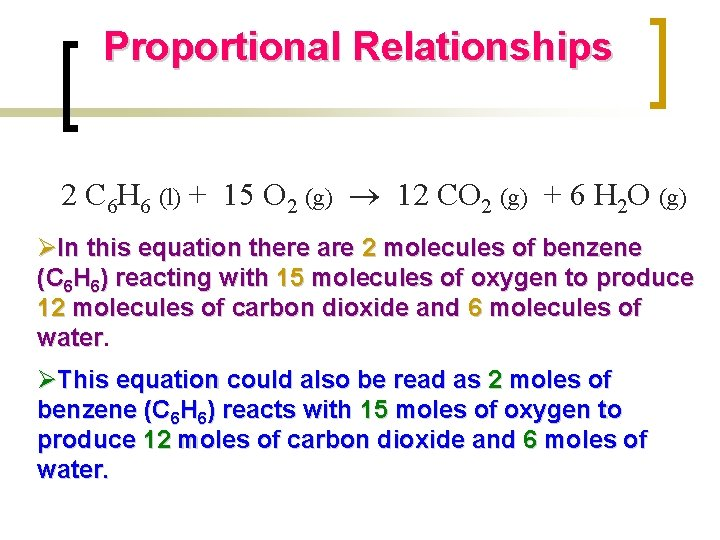Proportional Relationships 2 C 6 H 6 (l) + 15 O 2 (g) 12 CO 2 (g) + 6 H 2 O (g) ØIn this equation there are 2 molecules of benzene (C 6 H 6) reacting with 15 molecules of oxygen to produce 12 molecules of carbon dioxide and 6 molecules of water ØThis equation could also be read as 2 moles of benzene (C 6 H 6) reacts with 15 moles of oxygen to produce 12 moles of carbon dioxide and 6 moles of water.Proportional Relationships Since the relationship between the number of molecules and the number of moles present is 6. 02 x 1023, a common factor between all species involved in the equation, we can establish the MOLE RATIO between the species involved in a chemical reaction.Proportional Relationships b. Mole Ratio • indicated by coefficients in a balanced equation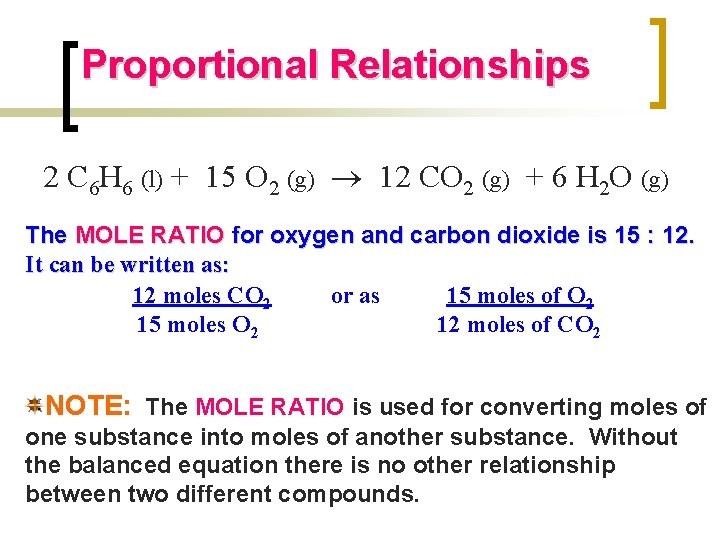Proportional Relationships 2 C 6 H 6 (l) + 15 O 2 (g) 12 CO 2 (g) + 6 H 2 O (g) The MOLE RATIO for oxygen and carbon dioxide is 15 : 12. It can be written as: 12 moles CO 2 or as 15 moles of O 2 15 moles O 2 12 moles of CO 2 NOTE: The MOLE RATIO is used for converting moles of one substance into moles of another substance. Without the balanced equation there is no other relationship between two different compounds.Stoichiometry Steps 1. Write a balanced equation. 2. Identify known & unknown. 3. Line up conversion factors. moles • Mole ratio • Molar mass moles grams • Avogadro’s # moles particles • Molar volume moles liters gas Core step in all stoichiometry problems!! 4. Calculate and check answer.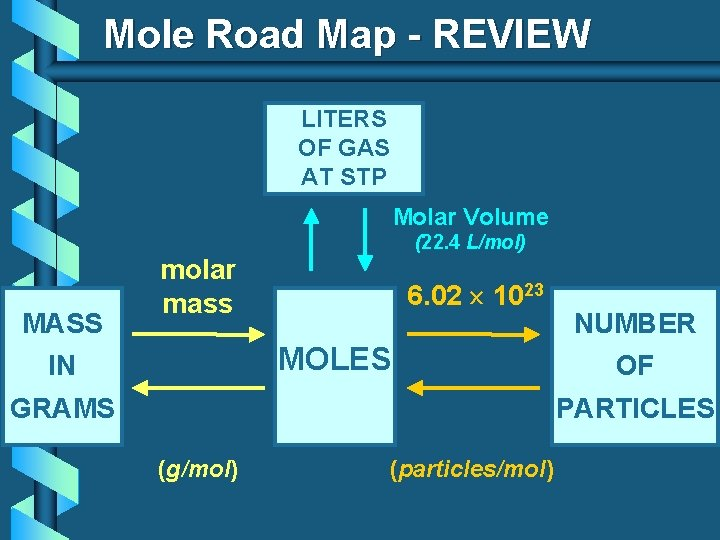Mole Road Map - REVIEW LITERS OF GAS AT STP Molar Volume MASS (22. 4 L/mol) molar mass 6. 02 1023 MOLES IN GRAMS NUMBER OF PARTICLES (g/mol) (particles/mol)Stoichiometry Island Diagram Mass 1 m ol e Known Unknown Substance A Substance B ) = ar m ol ar ol m as s Use coefficients from balanced chemical equation (g ) Volume 1 mole = 22. 4 L @ STP Mole (gases) s) Particles es t cl uni i t r pa ula 23 m 10 r x r fo 02 , o 6. les = u e ec ol ol m 1 s, m om at ( e 1 Mole ol m = s as (g Mass m m 1 mole = 22. 4 L @ STP Volume (gases) MOLE RATIO! 1 (a m to ol m e s, = m 6. ol 02 ec x ul 10 2 es 3 , o pa rf rti or cl m es ul a un its ) Particles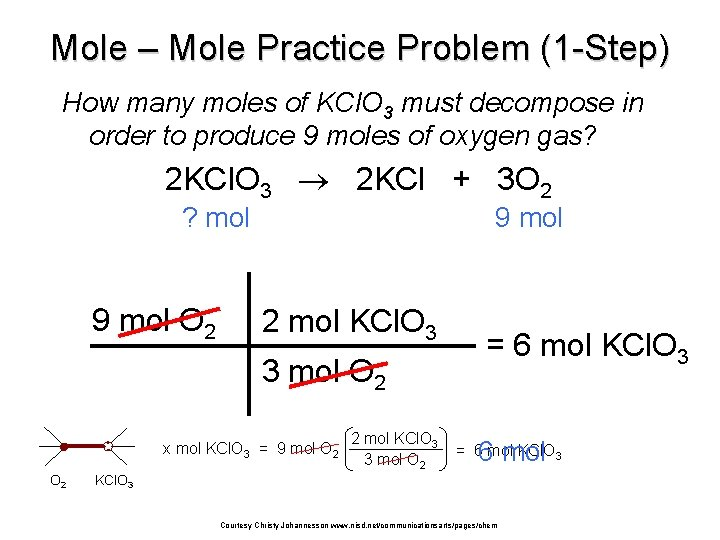Mole – Mole Practice Problem (1 -Step) How many moles of KCl. O 3 must decompose in order to produce 9 moles of oxygen gas? 2 KCl. O 3 2 KCl + 3 O 2 ? mol 9 mol O 2 9 mol 2 mol KCl. O 3 3 mol O 2 x mol KCl. O 3 = 9 mol O 2 2 mol KCl. O 3 3 mol O 2 = 6 mol KCl. O 3 6 mol = 6 mol KCl. O 3 Courtesy Christy Johannesson www. nisd. net/communicationsarts/pages/chem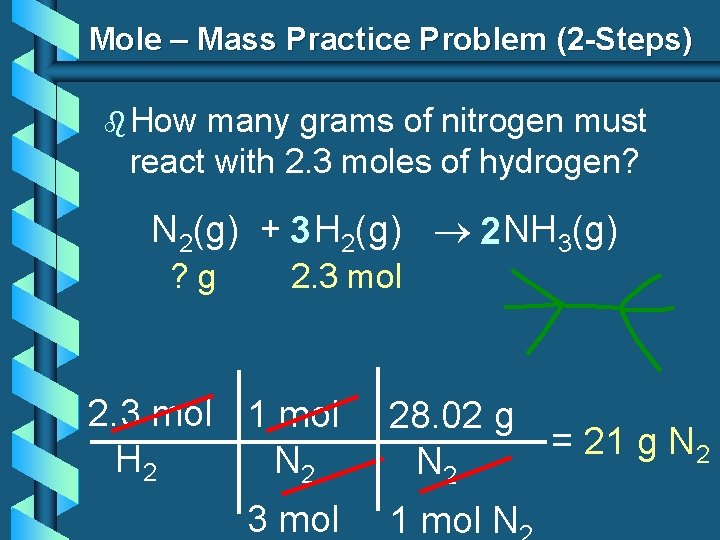Mole – Mass Practice Problem (2 -Steps) b How many grams of nitrogen must react with 2. 3 moles of hydrogen? N 2(g) + 3 H 2(g) 2 NH 3(g) ? g 2. 3 mol 1 mol H 2 N 2 3 mol 28. 02 g = 21 g N 2 1 mol N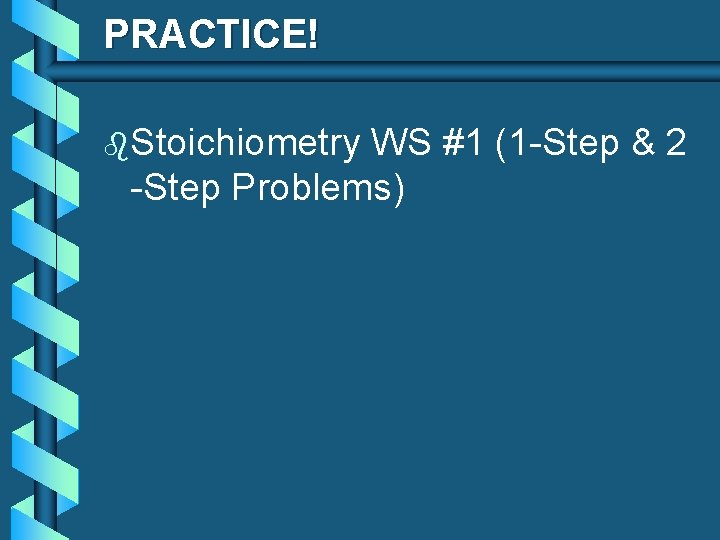PRACTICE! b. Stoichiometry WS #1 (1 -Step & 2 -Step Problems)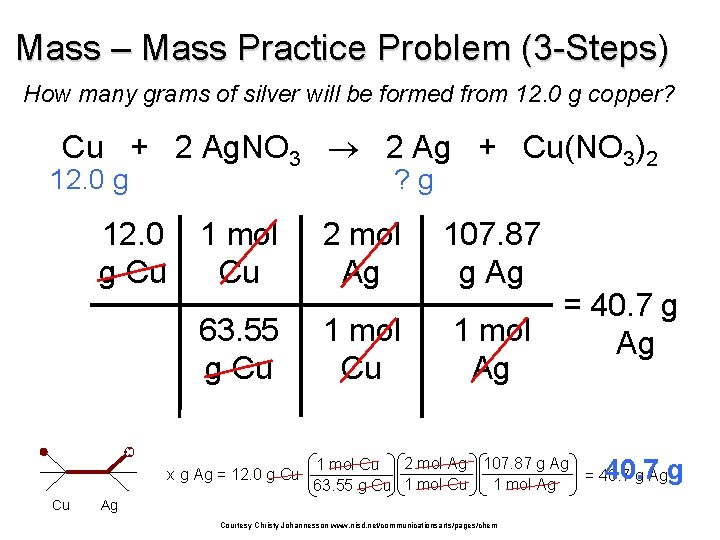Mass – Mass Practice Problem (3 -Steps) How many grams of silver will be formed from 12. 0 g copper? Cu + 2 Ag. NO 3 2 Ag + Cu(NO 3)2 12. 0 g Cu ? g 1 mol Cu 2 mol Ag 107. 87 g Ag 63. 55 g Cu 1 mol Ag x g Ag = 12. 0 g Cu Cu = 40. 7 g Ag 2 mol Ag 107. 87 g Ag 1 mol Cu 1 mol Ag 63. 55 g Cu 1 mol Cu Ag Courtesy Christy Johannesson www. nisd. net/communicationsarts/pages/chem 40. 7 g = 40. 7 g Ag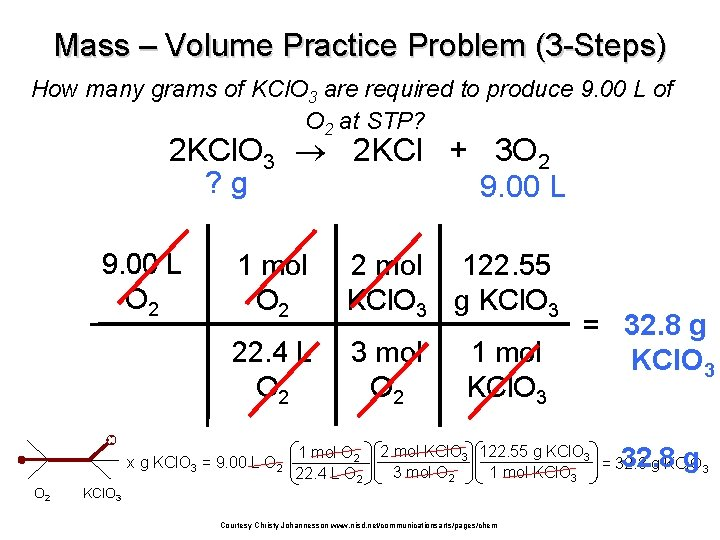Mass – Volume Practice Problem (3 -Steps) How many grams of KCl. O 3 are required to produce 9. 00 L of O 2 at STP? 2 KCl. O 3 2 KCl + 3 O 2 ? g 9. 00 L O 2 1 mol O 2 2 mol 122. 55 KCl. O 3 g KCl. O 3 22. 4 L O 2 3 mol O 2 x g KCl. O 3 = 9. 00 L O 2 1 mol O 2 22. 4 L O 2 1 mol KCl. O 3 = 32. 8 g KCl. O 3 32. 8 g 2 mol KCl. O 3 122. 55 g KCl. O 3 = 32. 8 g KCl. O 3 3 mol O 2 1 mol KCl. O 3 Courtesy Christy Johannesson www. nisd. net/communicationsarts/pages/chemStoichiometry Practice 2 KCl. O 3 2 KCl 500. g xg x L O 2 = 500. g KCl. O 3 122. 55 g KCl. O 3 x. L (196 g) 3 mol O 2 22. 4 L O 2 137 LLO 2 = 137 2 mol KCl. O 3 1 mol O 2 x g O 2 = 137 L O 2 1 mol O 2 32 g O 2 = 196 g O 2 22. 4 L O 2 1 mol O 2 x g KCl = 500. g KCl. O 3 1 mol KCl. O 3 + 3 O 2 KCl 1 mol KCl. O 3 122. 55 g KCl. O 3 2 mol KCl 74. 55 g KCl (304 gg)KCl = 304 2 mol KCl. O 3 1 mol KClBaking Soda Lab Na Na. HCO HCl HCO 3 + H Cl sodium bicarbonate + H 2 CO 3 hydrochloric acid sodium chloride baking soda table salt D (l) + H 2 O (g) heat CO 2 (g) actual yield ? g Na. HCO 3 + HCl 5 g excess D actual yield theoretical yield gas Na. Cl + H 2 O + CO 2 xg theoretical yield % yield = gas x 100 %PRACTICE! b. Stoichiometry Problems) WS #2 (3 -StepUnit 7 - Stoichiometry II. Stoichiometry in the Real World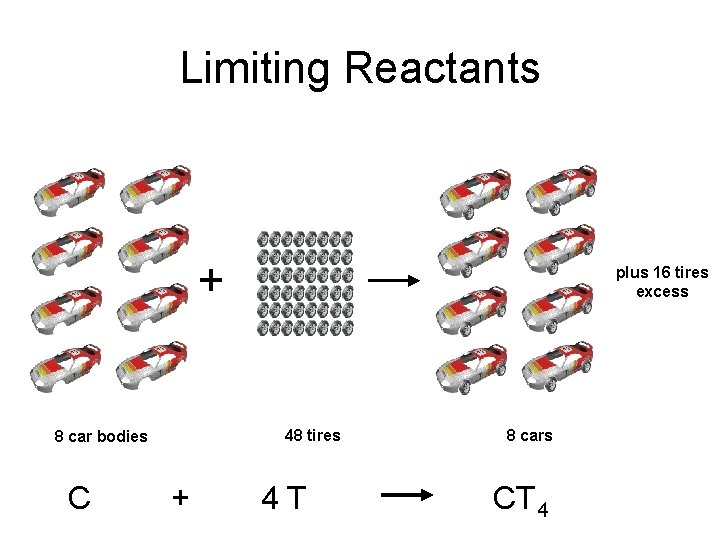Limiting Reactants + 48 tires 8 car bodies C plus 16 tires excess + 4 T 8 cars CT 4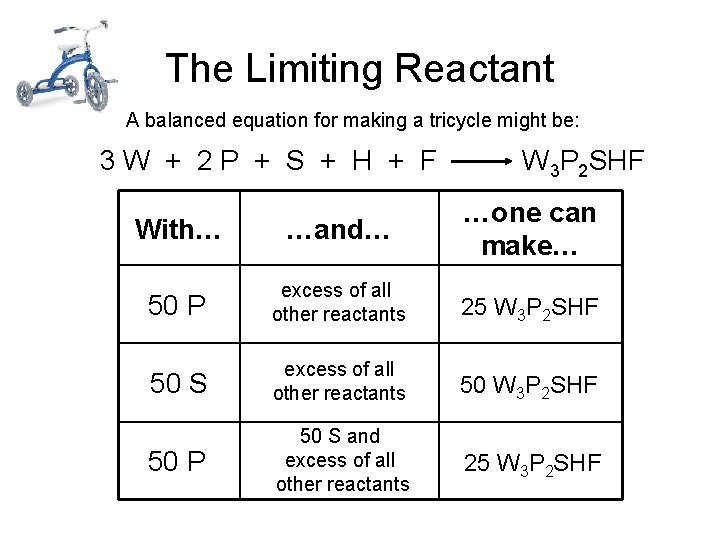The Limiting Reactant A balanced equation for making a tricycle might be: 3 W + 2 P + S + H + F W 3 P 2 SHF With… …and… …one can make… 50 P excess of all other reactants 25 W 3 P 2 SHF 50 S excess of all other reactants 50 W 3 P 2 SHF 50 P 50 S and excess of all other reactants 25 W 3 P 2 SHFLimiting Reactants b. Limiting Reactant • used up in a reaction • determines the amount of product b. Excess Reactant • what is left over after reaction • added to ensure that the other reactant is completely used upVisualizing Limiting Reactant 2 H 2 + O 2 7 0 8 3 4 2 6 5 mole H 2 1 ___ 2. 5 1. 5 4. 5 3. 5 1 5 3 mole O 2 2 4 ___ Limiting Reactant Excess 2 H 2 O 1 5 8 0 4 6 2 3 mole H 2 O 7 ___ Limiting reactant determines amount of product.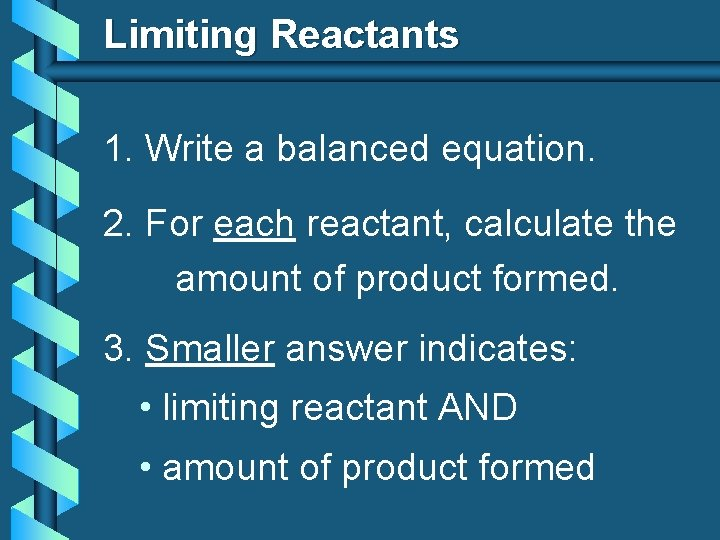Limiting Reactants 1. Write a balanced equation. 2. For each reactant, calculate the amount of product formed. 3. Smaller answer indicates: • limiting reactant AND • amount of product formed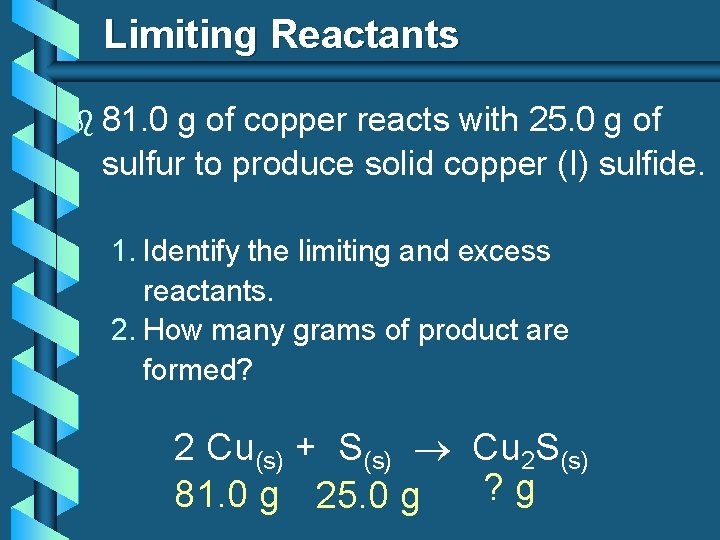Limiting Reactants b 81. 0 g of copper reacts with 25. 0 g of sulfur to produce solid copper (I) sulfide. 1. Identify the limiting and excess reactants. 2. How many grams of product are formed? 2 Cu(s) + S(s) Cu 2 S(s) ? g 81. 0 g 25. 0 g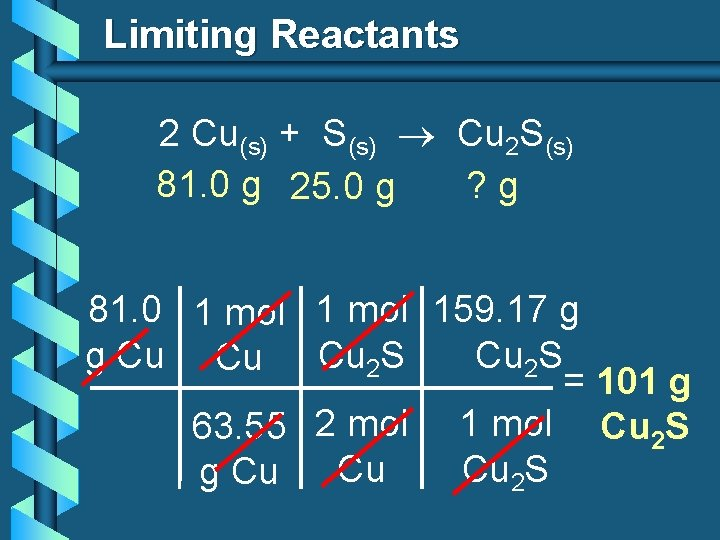Limiting Reactants 2 Cu(s) + S(s) Cu 2 S(s) 81. 0 g 25. 0 g ? g 81. 0 1 mol 159. 17 g g Cu Cu Cu 2 S = 101 g 63. 55 2 mol 1 mol Cu 2 S Cu Cu 2 S g CuLimiting Reactants 2 Cu(s) + S(s) Cu 2 S(s) 81. 0 g 25. 0 g ? g 25. 0 1 mol 159. 17 g g. S Cu 2 S = 124 g 32. 07 1 mol Cu 2 S S g. S Cu 2 S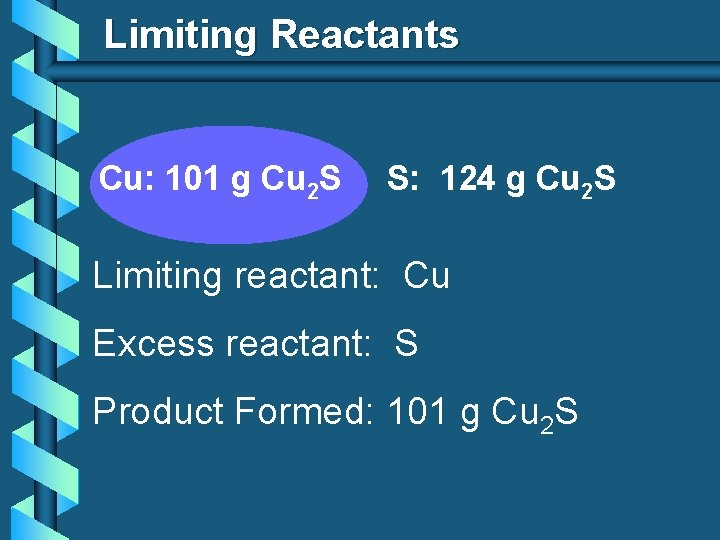Limiting Reactants Cu: 101 g Cu 2 S S: 124 g Cu 2 S Limiting reactant: Cu Excess reactant: S Product Formed: 101 g Cu 2 S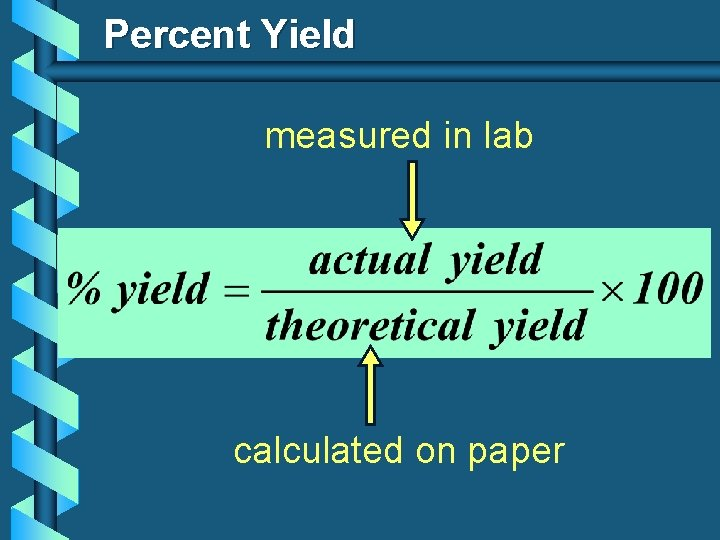Percent Yield measured in lab calculated on paper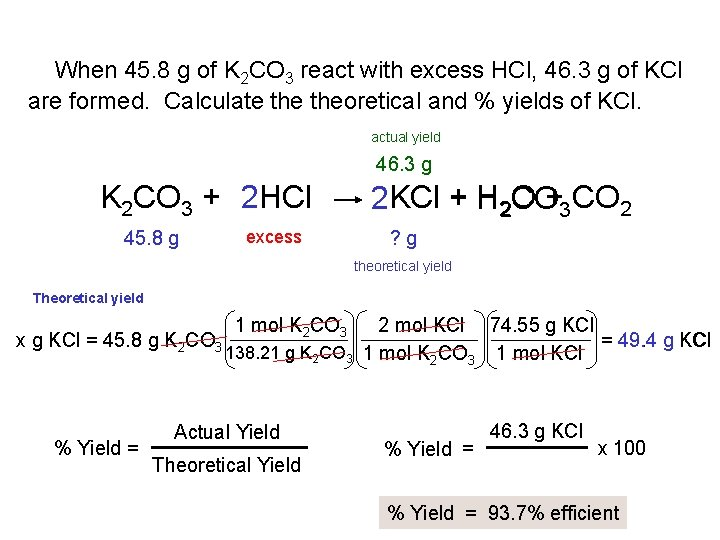When 45. 8 g of K 2 CO 3 react with excess HCl, 46. 3 g of KCl are formed. Calculate theoretical and % yields of KCl. actual yield 46. 3 g K 2 CO 3 + 2 HCl 45. 8 g excess 2 KCl + H 2 O CO+3 CO 2 ? g theoretical yield Theoretical yield x g KCl = 45. 8 g K 2 CO 3 % Yield = 1 mol K 2 CO 3 2 mol KCl 74. 55 g KCl = 49. 4 g KCl 138. 21 g K 2 CO 3 1 mol KCl Actual Yield Theoretical Yield % Yield = 46. 3 g KCl x 100 % Yield = 93. 7% efficient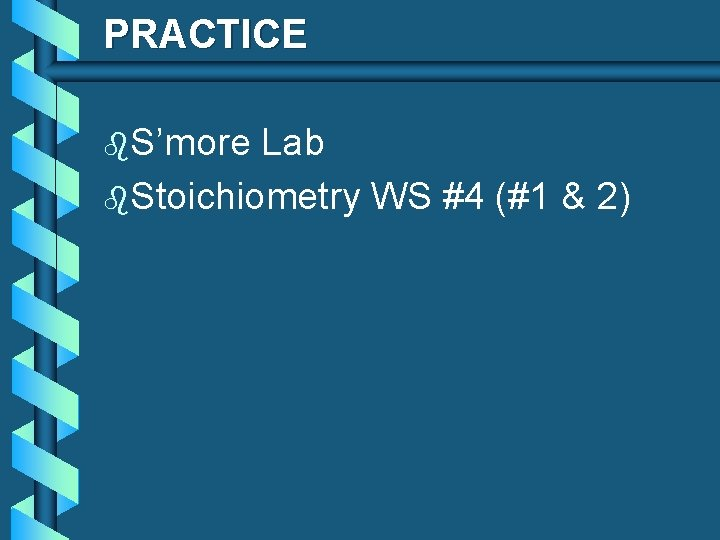PRACTICE b. S’more Lab b. Stoichiometry WS #4 (#1 & 2)